A Guide to Interpreting CPC Products

6-10 Day Outlooks

8-14 Day Outlooks

6-10 Day and 8-14 Day Rules

Weeks 3-4 Outlook

Weeks 3-4 Rules

One Month Outlook

One Month and Three Month Rules

Terciles Visualization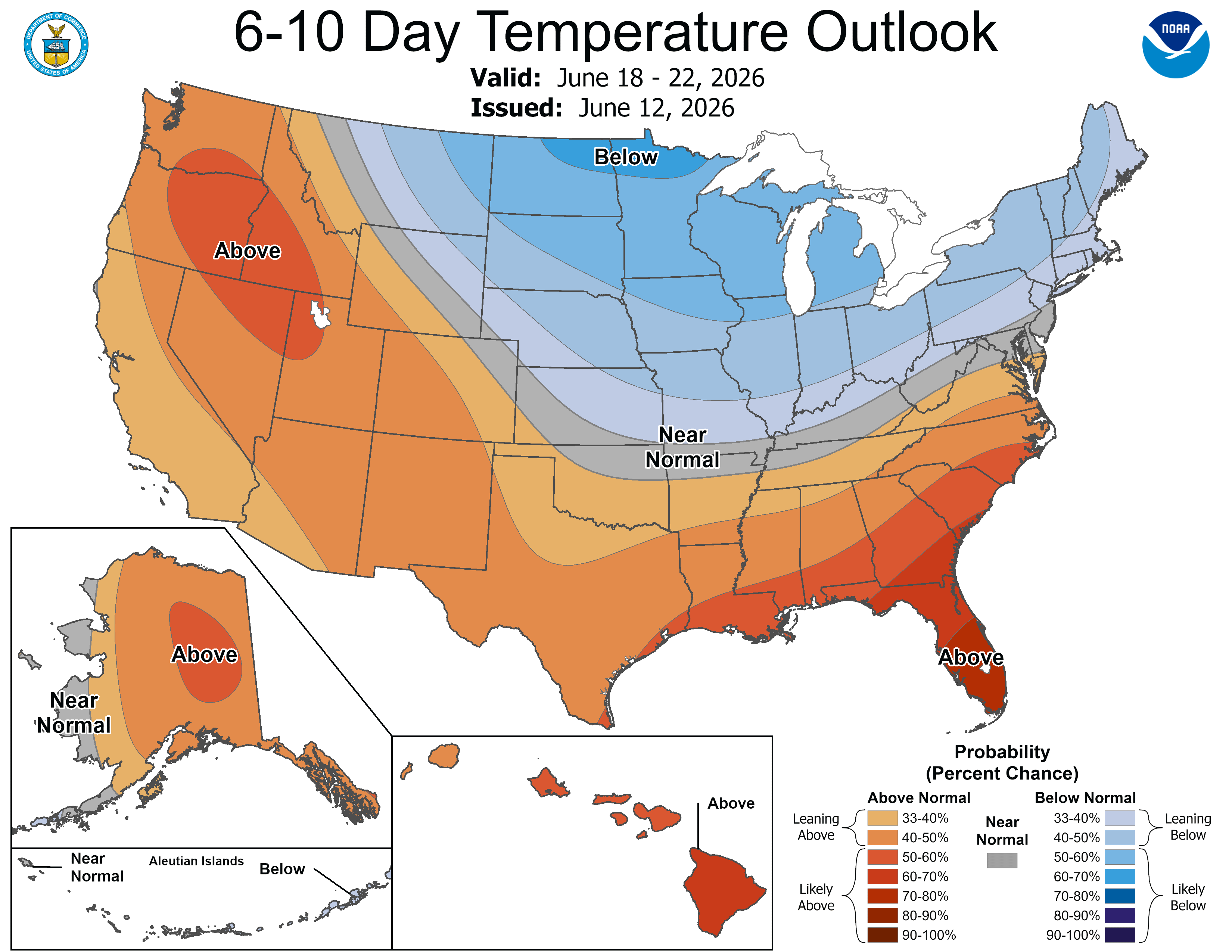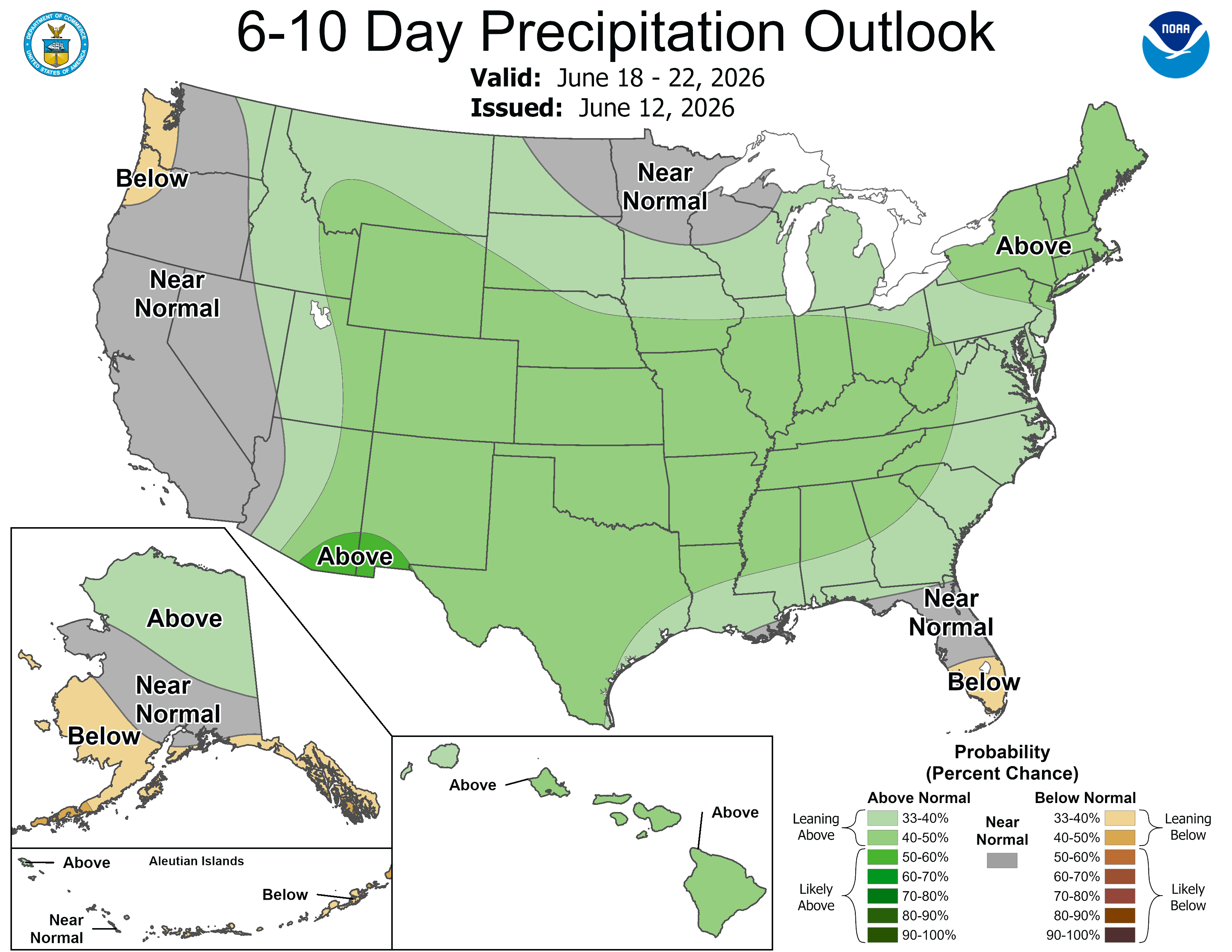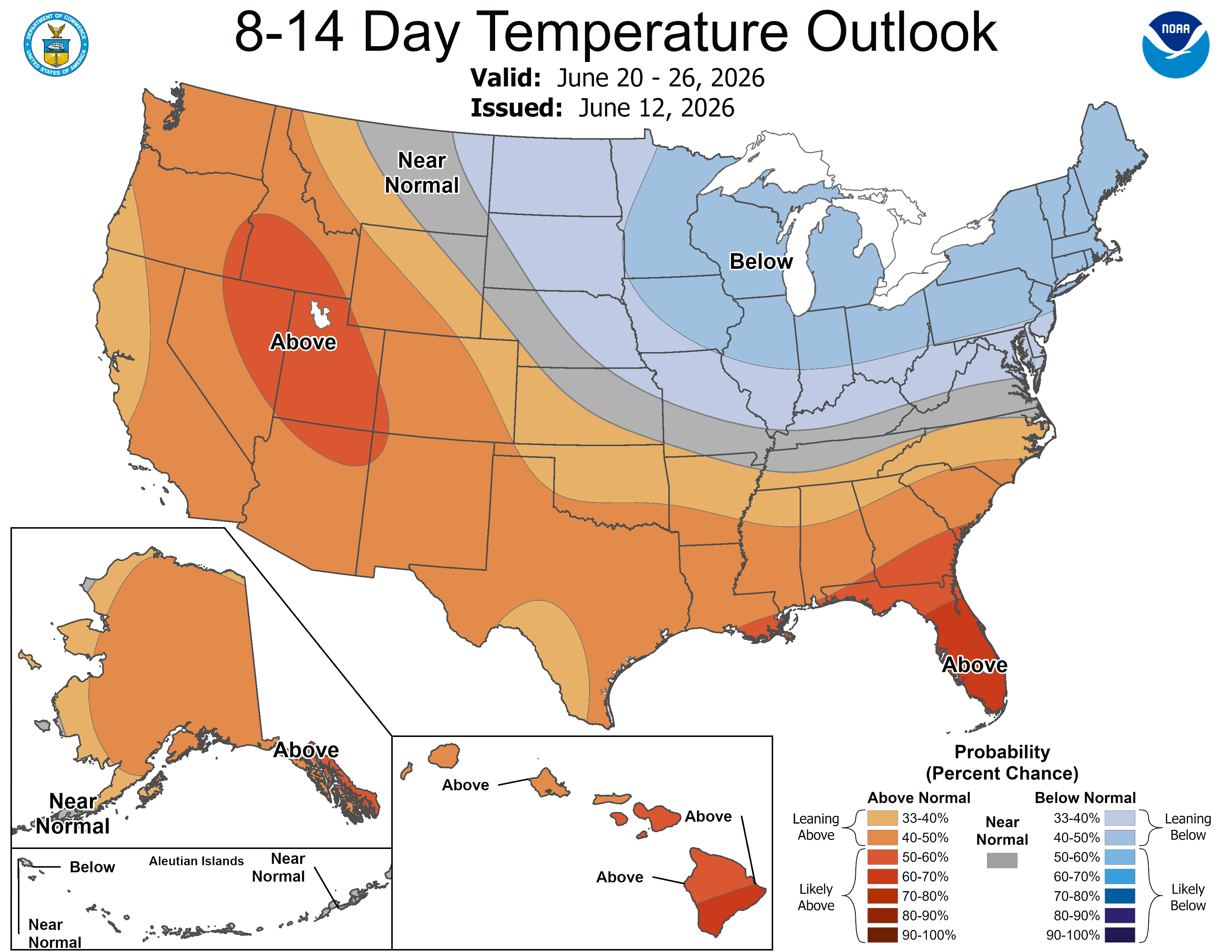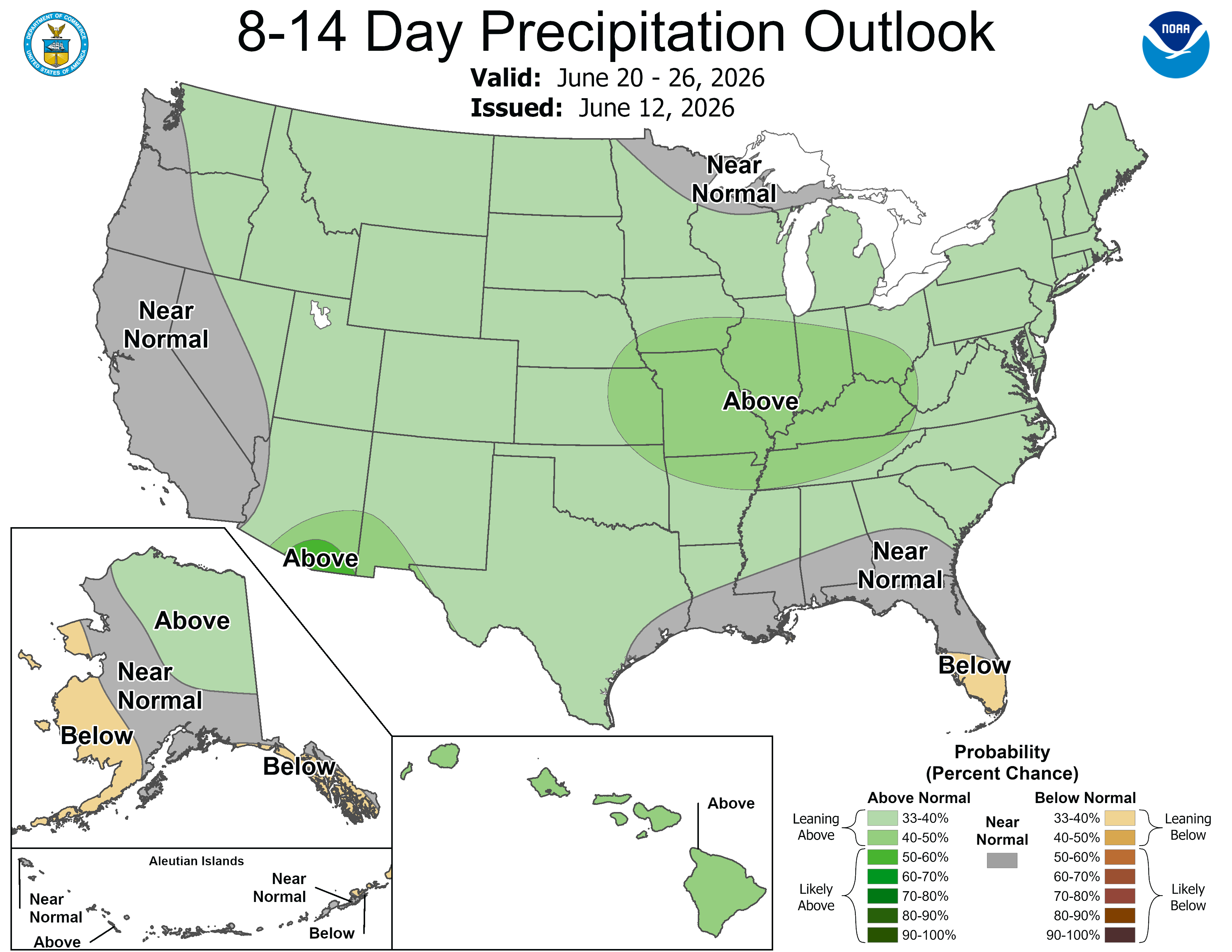Weeks 3-4 Outlook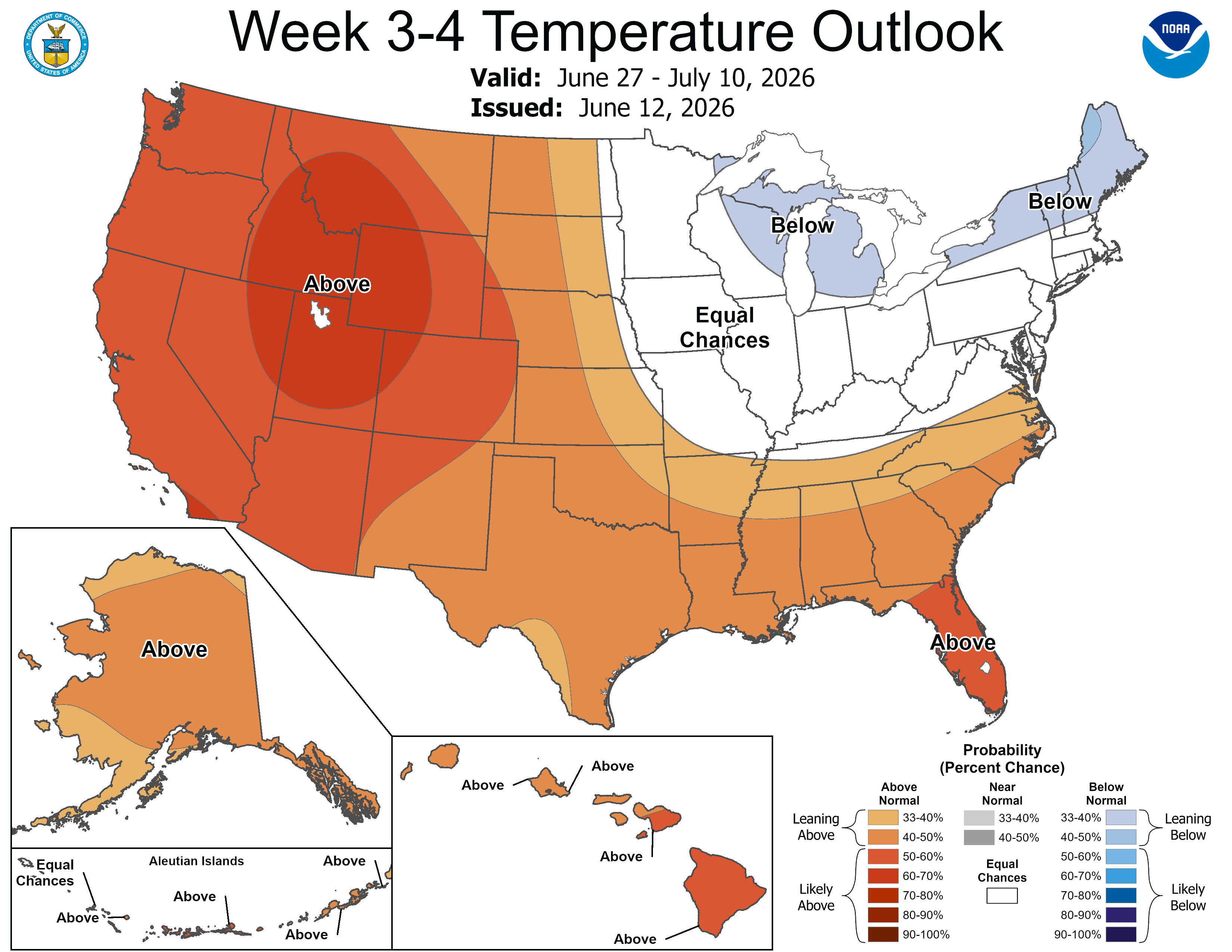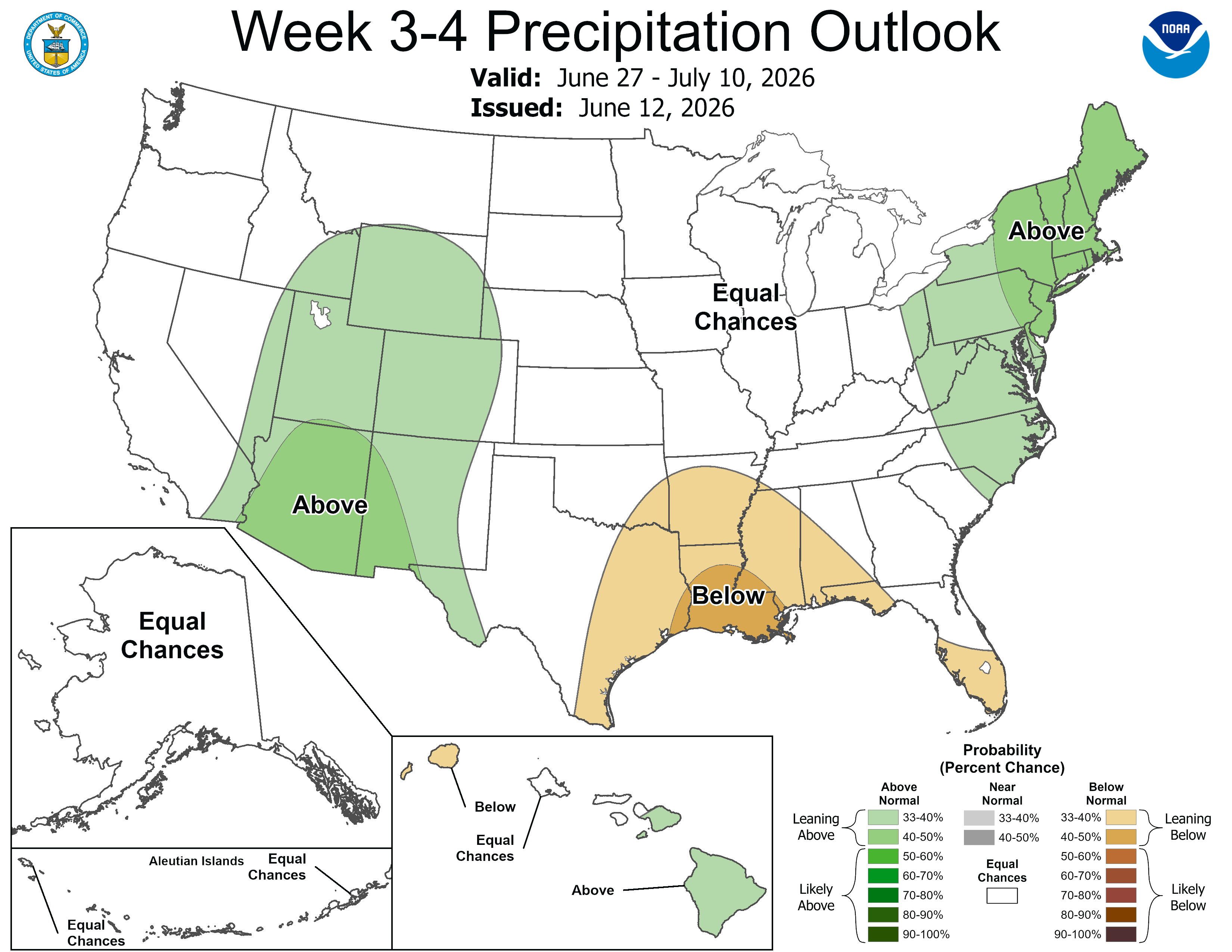One Month Outlook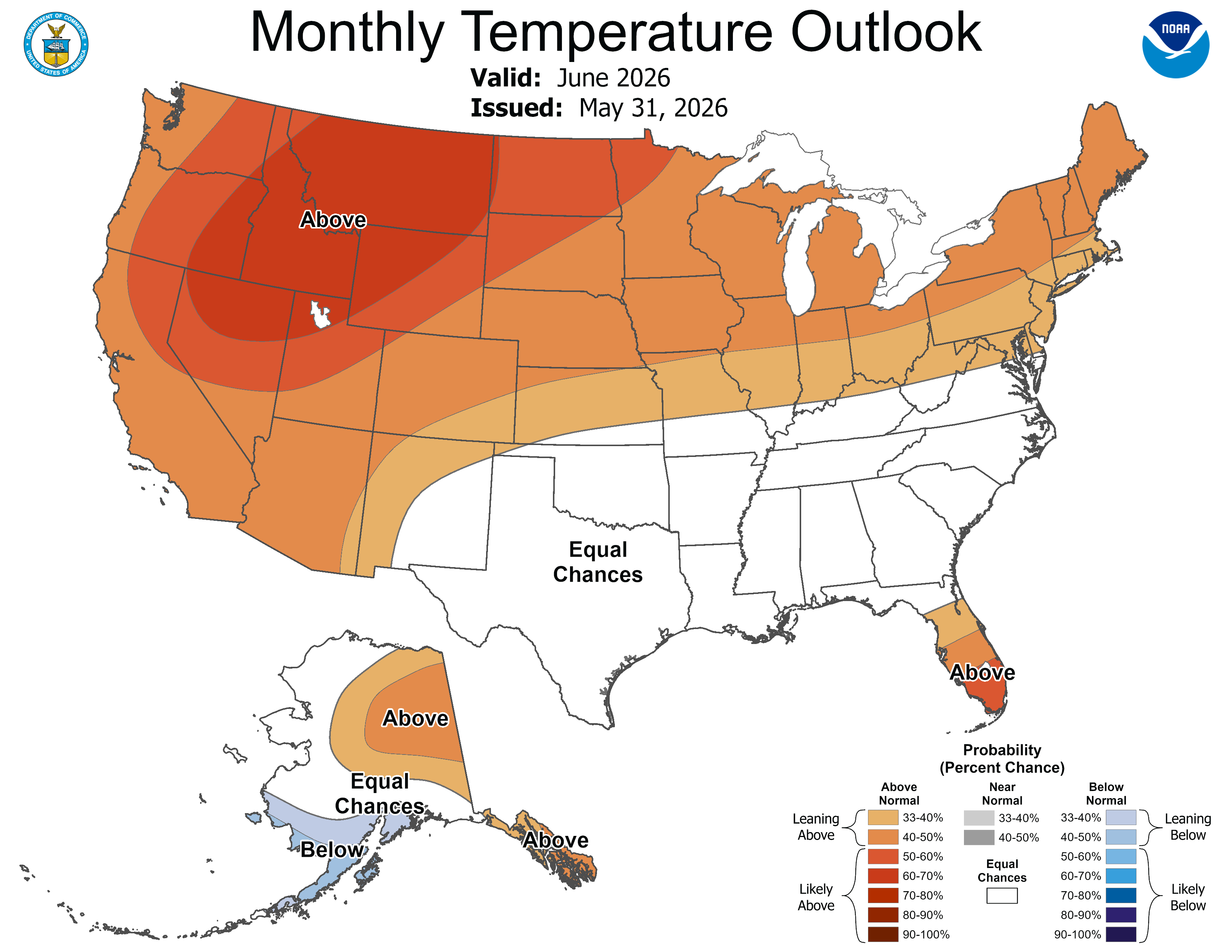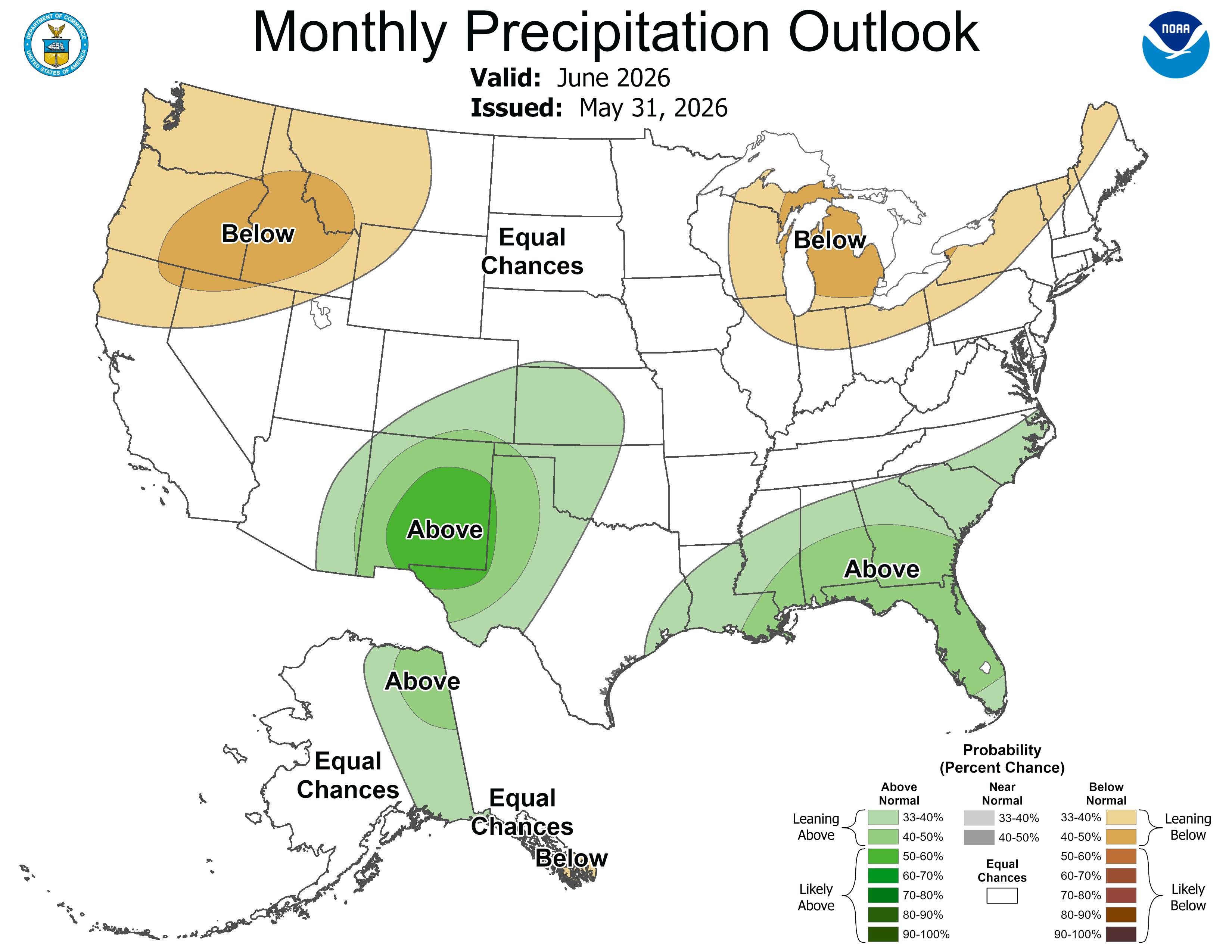Three Month Outlook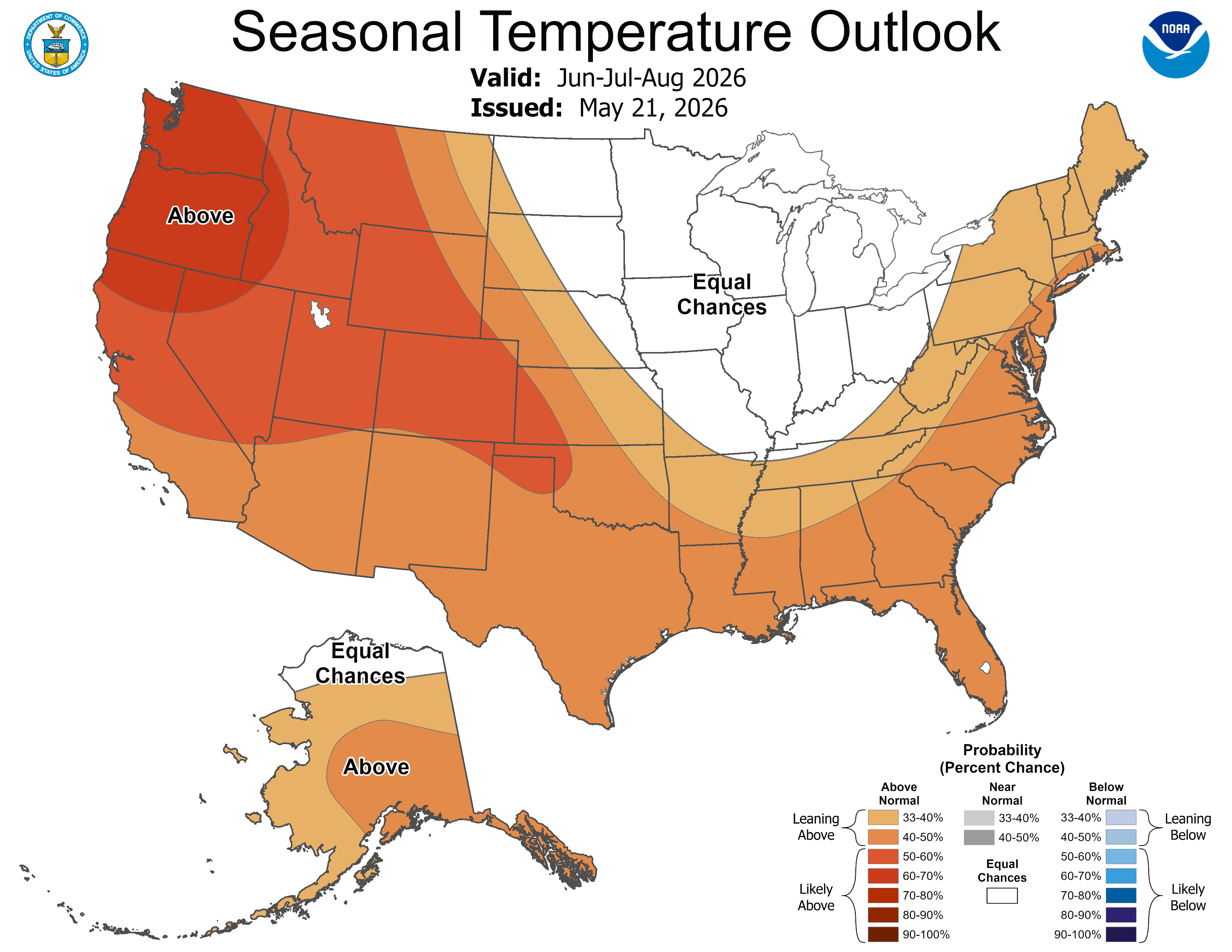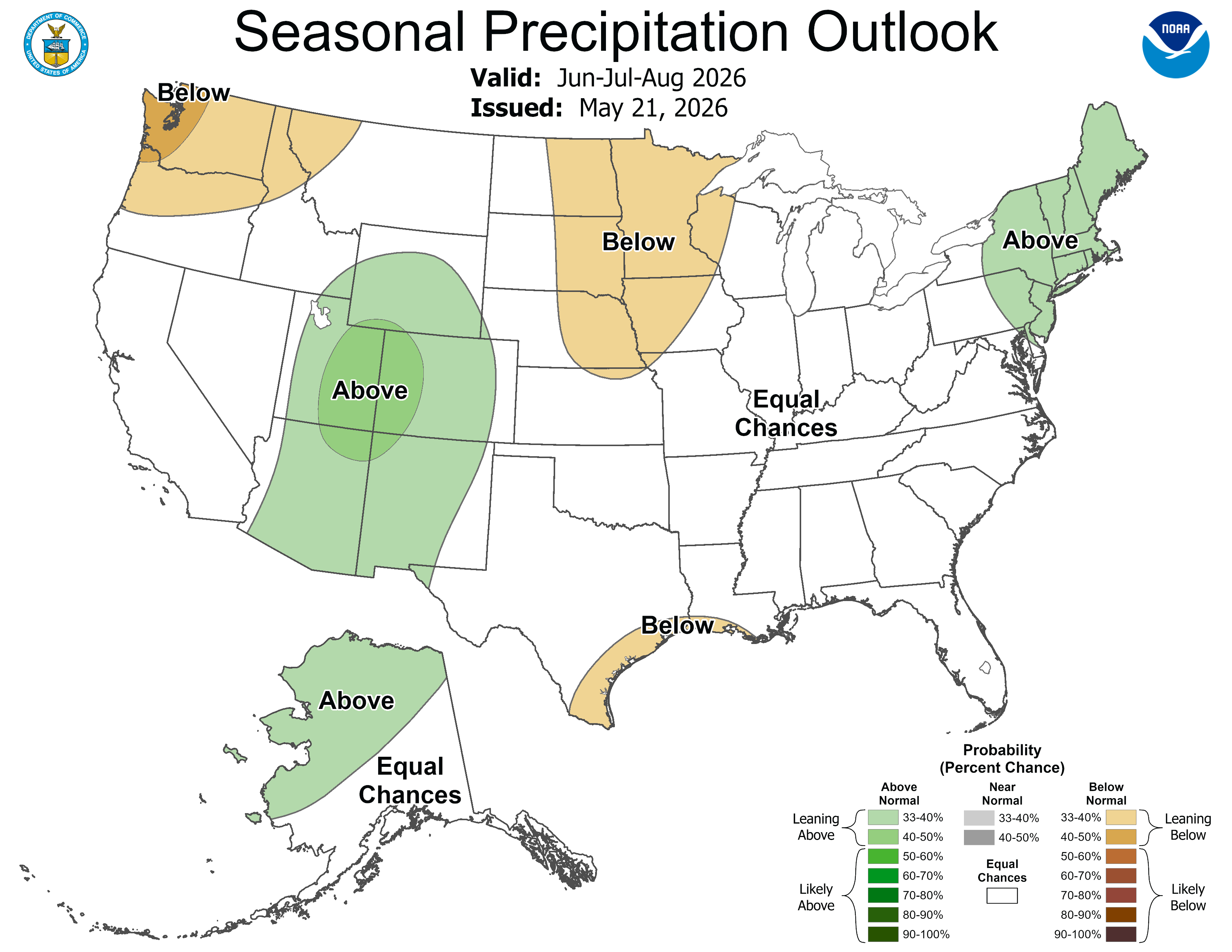6-10 Day and 8-14 Day Rules

Temperature: CPC's 6-10 day and 8-14 day temperature maps show the probability of the observed temperature (the average of the daily mean temperature of all the days in the period) will be in one of three different categories: above normalnear normal and below normal. The daily mean temperature is the 24hr average of all temperature observations for a given station on a given day. The 6-10 Day Average and 8-14 Day Average are the average of all the daily mean temperatures within either the 6-10 day period or 8-14 day period.

Daily Mean Temperature = Sum of the Temperature Observation Values/Number of Temperature Observations in a 24hr period

6-10 Day Average = Sum of the Daily Mean Temperature Values (Days 6-10)/Number of Days in Period

8-14 Day Average = Sum of the Daily Mean Temperature Values (Days 8-14)/Number of Days in Period

These maps show the probability of the 6-10 Day and 8-14 Day Average Temperature being above, near and below the 1991-2020 climate normal for the period.

Precipitation: CPC 6-10 day and 8-14 day precipitation maps show the probability that the observed precipitation (the total precipitation of all days in the period) will be in one of three different categories: above normalnear normal and below normal.

These maps show the probability of the 6-10 Day and 8-14 Day Total Precipitation being above, near and below the 1991-2020 climate normal for the period.

Rules and Examples of Interpreting CPC's 6-10 Day and 8-14 Day Probabilistic Outlooks

1) If the probability of "above" or "below" temperature and/or precipitation is between 33.3% and 63.4%:

- Probability of the opposite category declines by the difference between the higher probability category and 33.3%

- Probability of the middle category remains at 33.3%.

Example 1: CPC's outlook shows a 40% probability of above normal temperatures during the 6-10 day period.

Probability of Above Normal Temperatures = 40%

Probability of Near Normal Temperatures = 33.3%

Probability of Below Normal Temperatures = 100% - [40% + 33.3%] = 100% - 73.3% = 26.7%

2) If the probability of "above" or "below" temperature/precipitation is greater than 63.4%

- Probability of the opposite category reaches the minimum allowed value of 3.3% (it does not matter if the favored category is 70%, 80% or 90% as long as it is above 63.4% the opposite category will be 3.3% every time).

- Probability of the middle category (near normaldecreases by the sum of "above" and "below."

***The probability of the non-favored category is NEVER zero***

Example 2: CPC's outlook shows an 80% chance of below normal precipitation during the 6-10 day period.

Probability of Above Normal Precipitation = 3.3%

Probability of Near Normal Precipitation = 100% - [80% + 3.3%] = 100% - 83.3% = 16.7%

Probability of Below Normal Precipitation = 80%

3) If the probability of near normal is greater than 33.3%

- Probability of each of the other categories decreases by half of the amount that the middle category exceeds 33.3%.

Example 3: CPC's outlook shows a 35% chance of near normal temperatures during the 6-10 day period.

Probability of Near Normal Temperatures = 35%

Probability of Above Normal Temperatures = 35% - 33.3% = 1.7% ---> 1.7%/2 = 0.85% ---> 33.3% - 0.85% = 32.45% (~32.5%)

Probability of Below Normal Temperatures = 35% - 33.3% = 1.7% ---> 1.7%/2 = 0.85% ---> 33.3% - 0.85% = 32.45% (~32.5%)

Weeks 3-4 Rules

Weeks 3-4 is the only category that has no "near normal" category. Weeks 3-4 has above normal, below normal and equal chances categories.

Since there is no "near normal" category, the rules are more simple here.

Daily Mean Temperature = Sum of the Temperature Observation Values/Number of Temperature Observations in a 24hr period

Weeks 3-4 Average = Sum of the Daily Mean Temperature Values (Over the Weeks 3-4 Period)/Number of Days in Period

Example 1: CPC's outlook shows a 60% chance of above normal temperatures in Weeks 3-4.

Probability of Above Normal Temperatures = 60%

Probability of Below Normal Temperatures = 40%

Example 2: CPC's outlook shows an 80% chance of below normal precipitation in Weeks 3-4.

Probability of Above Normal Precipitation = 20%

Probability of Below Normal Precipitation = 80%

Example 3: CPC's Outlook shows equal chances of above or below normal temperatures in Weeks 3-4.

Probability of Above Normal Temperatures = 50%

Probability of Below Normal Temperatures = 50%.

One Month and Three Month Rules

Temperature: CPC's One Month and Three Month temperature maps show the probability of the observed temperature (the average of the daily mean temperature of all the days in the period) will be in one of three different categories: above normalnear normal and below normal. The daily mean temperature is the 24hr average of all temperature observations for a given station on a given day. The One Month Average and Three Month Average are the average of all the daily mean temperatures within either the One Month or Three Month period.

Daily Mean Temperature = Sum of the Temperature Observation Values/Number of Temperature Observations in a 24hr period

One Month Average = Sum of the Daily Mean Temperature Values (Over a 1-Month Period)/Number of Days in Period

Three Month Average = Sum of the Daily Mean Temperature Values (Over a 3-Month Period)/Number of Days in Period

Precipitation: CPC's One Month and Three Month precipitation maps show the probability that the observed precipitation (the total precipitation of all days in the period) will be in one of three different categories: above normalnear normal and below normal.

Rules and Examples of Interpreting CPC's One Month and Three Month Probabilistic Outlooks

1) If the probability of "above" or "below" temperature and/or precipitation is between 33.3% and 63.4%:

- Probability of the opposite category declines by the difference between the higher probability category and 33.3%

- Probability of the middle category remains at 33.3%.

Example 1: CPC's outlook shows a 40% probability of above normal temperatures during the One Month period.

Probability of Above Normal Temperatures = 40%

Probability of Near Normal Temperatures = 33.3%

Probability of Below Normal Temperatures = 100% - [40% + 33.3%] = 100% - 73.3% = 26.7%

2) If the probability of "above" or "below" temperature/precipitation is greater than 63.4%

- Probability of the opposite category reaches the minimum allowed value of 3.3% (it does not matter if the favored category is 70%, 80% or 90% as long as it is above 63.4% the opposite category will be 3.3% every time).

- Probability of the middle category (near normaldecreases by the sum of "above" and "below."

***The probability of the non-favored category is NEVER zero***

Example 2: CPC's outlook shows an 80% chance of below normal precipitation during the Three Month period.

Probability of Above Normal Precipitation = 3.3%

Probability of Near Normal Precipitation = 100% - [80% + 3.3%] = 100% - 83.3% = 16.7%

Probability of Below Normal Precipitation = 80%

3) If there are equal chances of above, below or near normal temperatures/precipitation:

- The probability remains at 33.3% for all three categories (above normal, near normal and below normal).

- Equal chances means that there is an equal chance of the odds tilting in any direction with no single direction being favored.

Example 4: CPC's outlook shows "equal chances" of above normal, near normal or below normal temperatures during the Three Month period.

Probability of Above Normal Temperatures = 33.3%

Probability of Near Normal Temperatures = 33.3%

Probability of Below Normal Temperatures = 33.3%

4) If the probability of near normal is greater than 33.3%

- Probability of each of the other categories decreases by half of the amount that the middle category exceeds 33.3%.

Example 4: CPC's outlook shows a 35% chance of near normal temperatures during the One Month period.

Probability of Near Normal Temperatures = 35%

Probability of Above Normal Temperatures = 35% - 33.3% = 1.7% ---> 1.7%/2 = 0.85% ---> 33.3% - 0.85% = 32.45% (~32.5%)

Probability of Below Normal Temperatures = 35% - 33.3% = 1.7% ---> 1.7%/2 = 0.85% ---> 33.3% - 0.85% = 32.45% (~32.5%)

***The near-normal category exists for the One Month and Three Month Outlooks. However it is RARELY used at these timescales.***

Tercile Examples via Visualization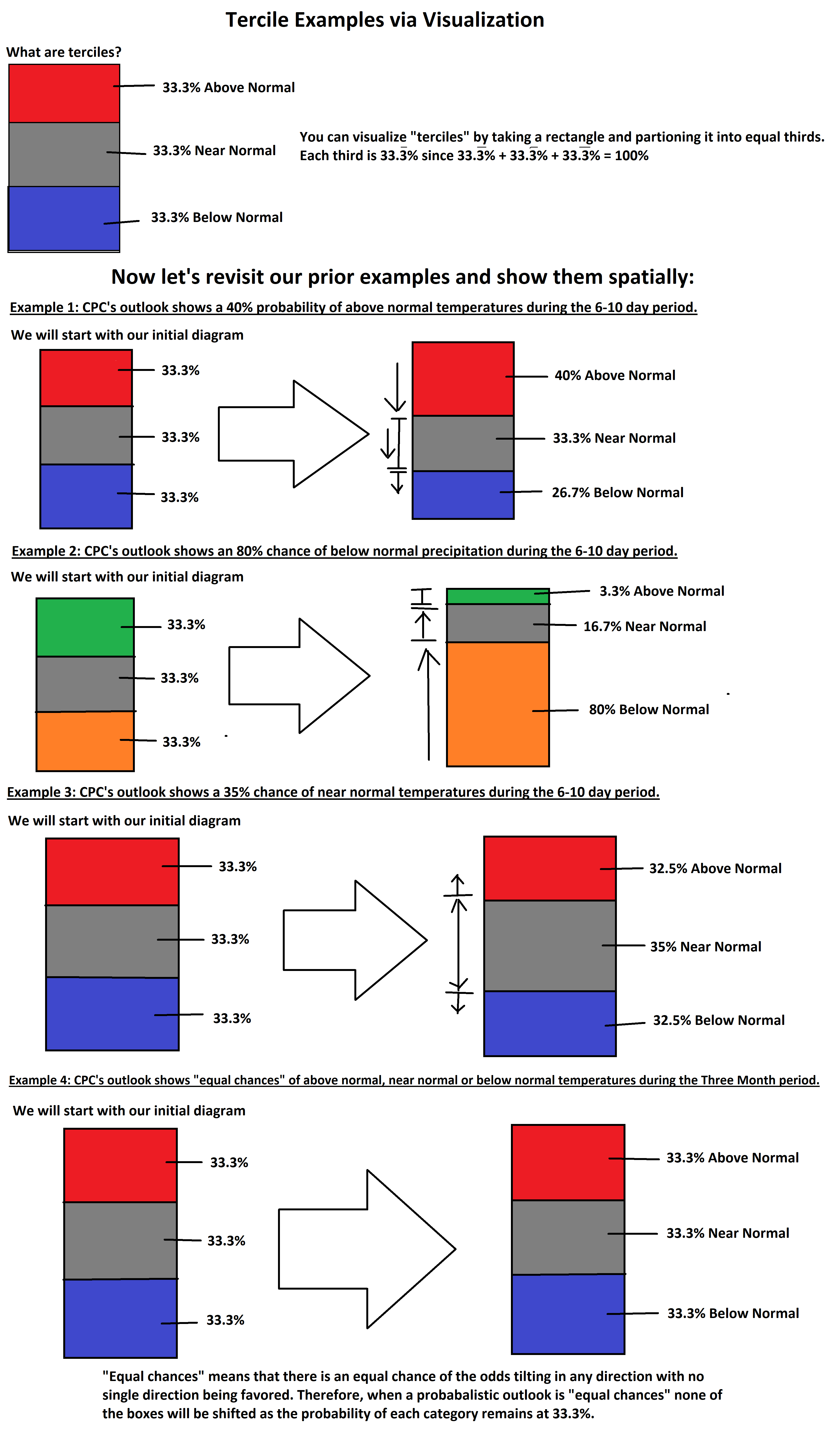There are a few things the maps do not tell us

1) Does not tell us if the favored category is possible on any given days or weeks in the period

2) Does not tell us if the maximum or minimum temperatures lean more strongly into the favored category.

3) Does not give us the exact probability of any one point on the map.

4) Does not tell us that particular temperatures will happen or that we can expect those temperatures.

5) Does not tell us anything about the precipitation-type (i.e. snow vs. rain).

6) Does not tell us that any area will be wetter or drier than any other

7) Does not tell us that particular precipitation totals will happen or that we can expect that precipitation

***These are probabilistic outlooks which means these products only tell us which way the odds tilt.***

Composite Outlook

Composite Outlook Rules

Hazardous Temperatures Outlook

Hazardous Temperatures Outlook Rules

Heavy Precipitation Outlook

Heavy Precipitation Outlook Rules

Heavy Snow Outlook

Heavy Snow Outlook Rules

High Winds Outlook

High Winds Outlook Rules

Rapid Onset Drought Methodology ***Experimental***

Composite Outlook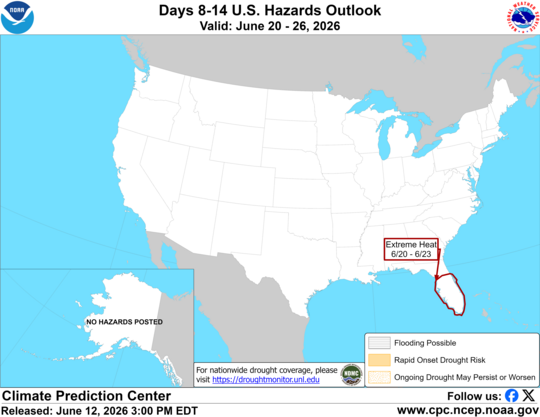Hazardous Temperatures Outlook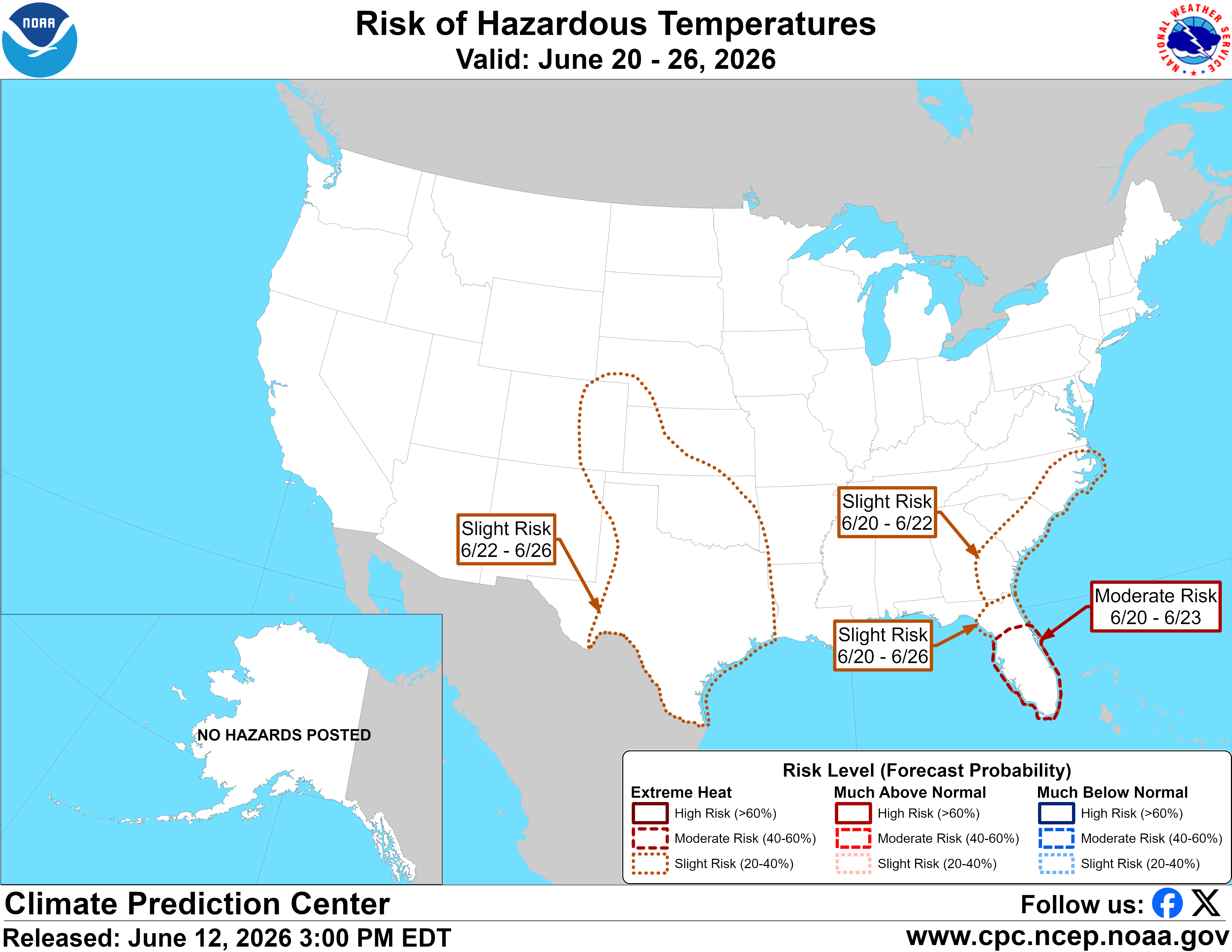Heavy Precipitation Outlook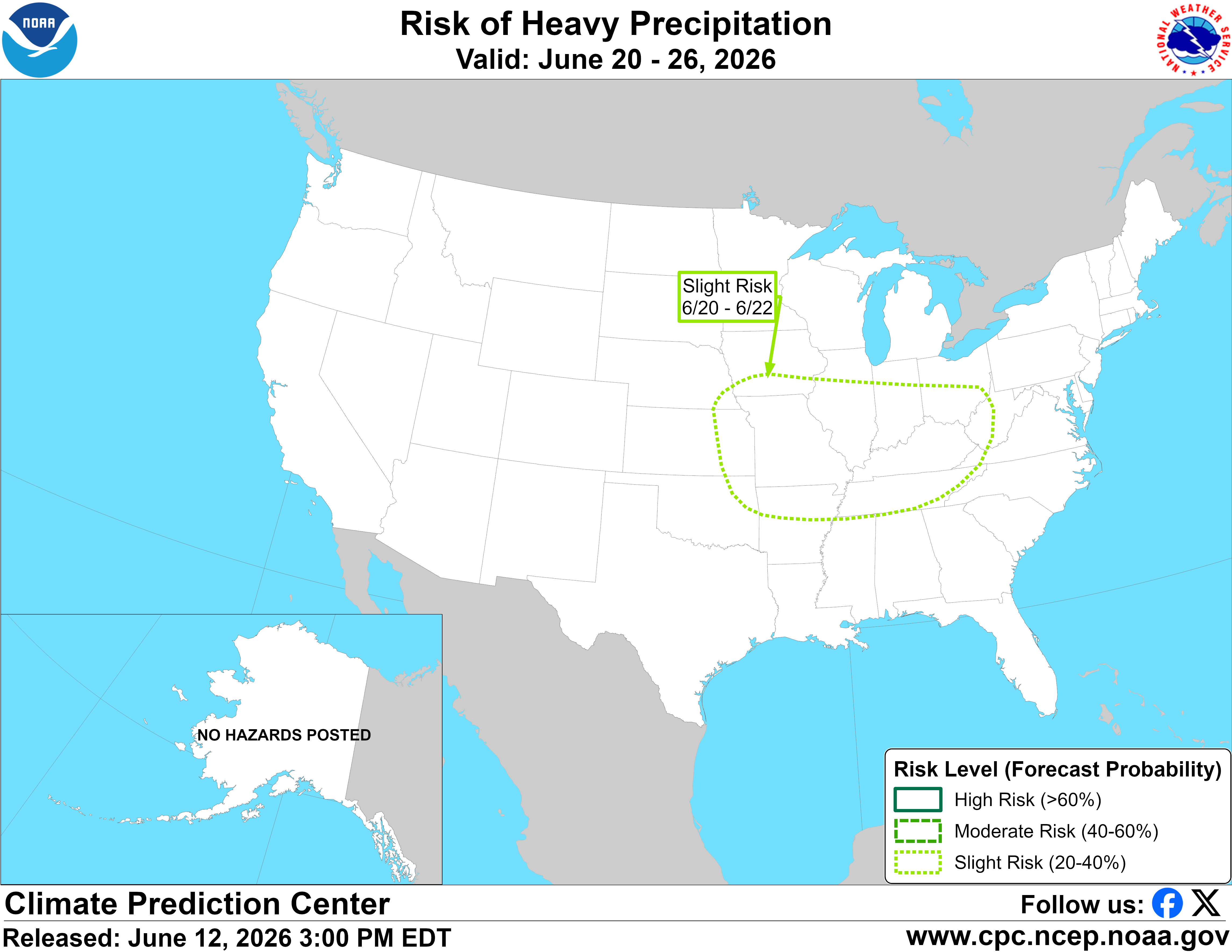Heavy Snow Outlook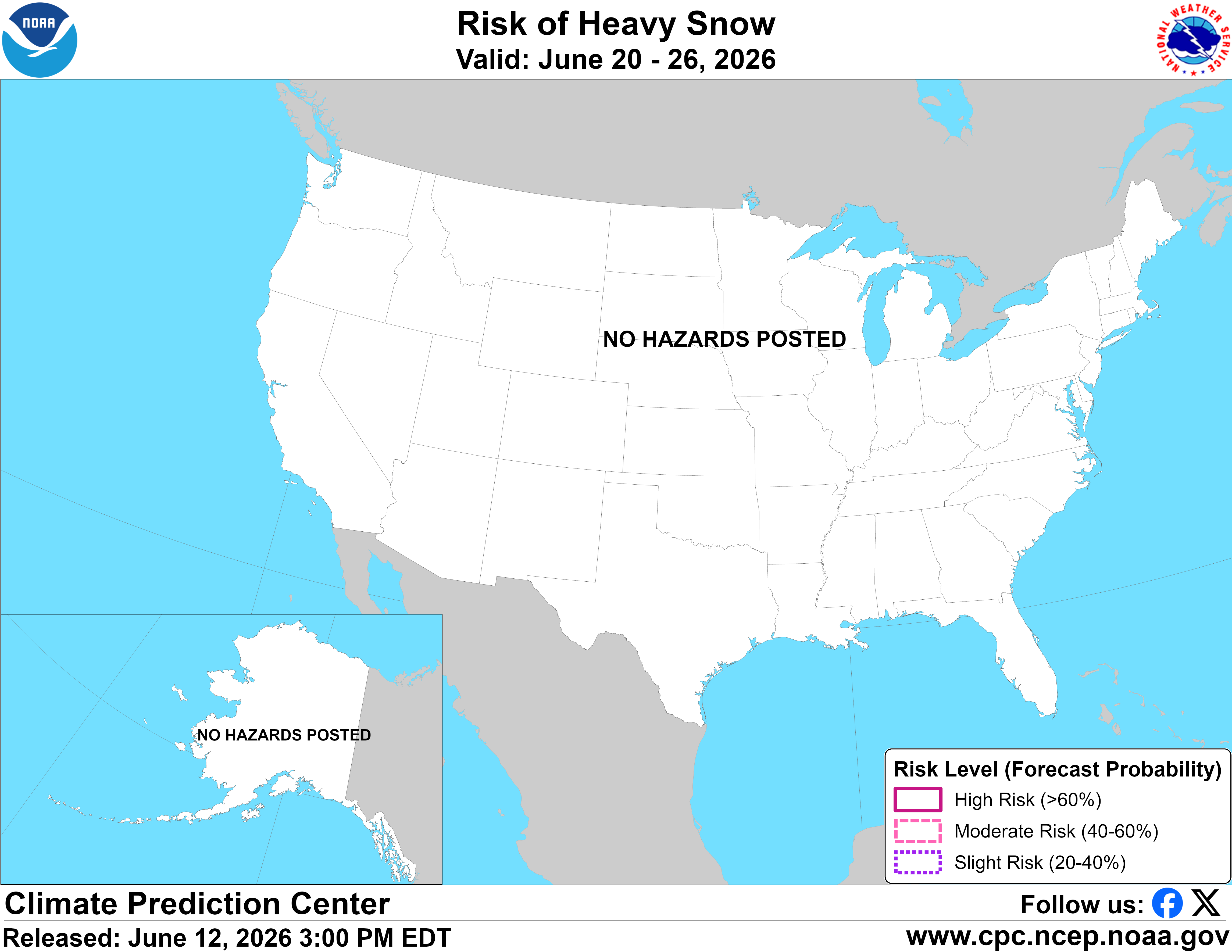High Winds Outlook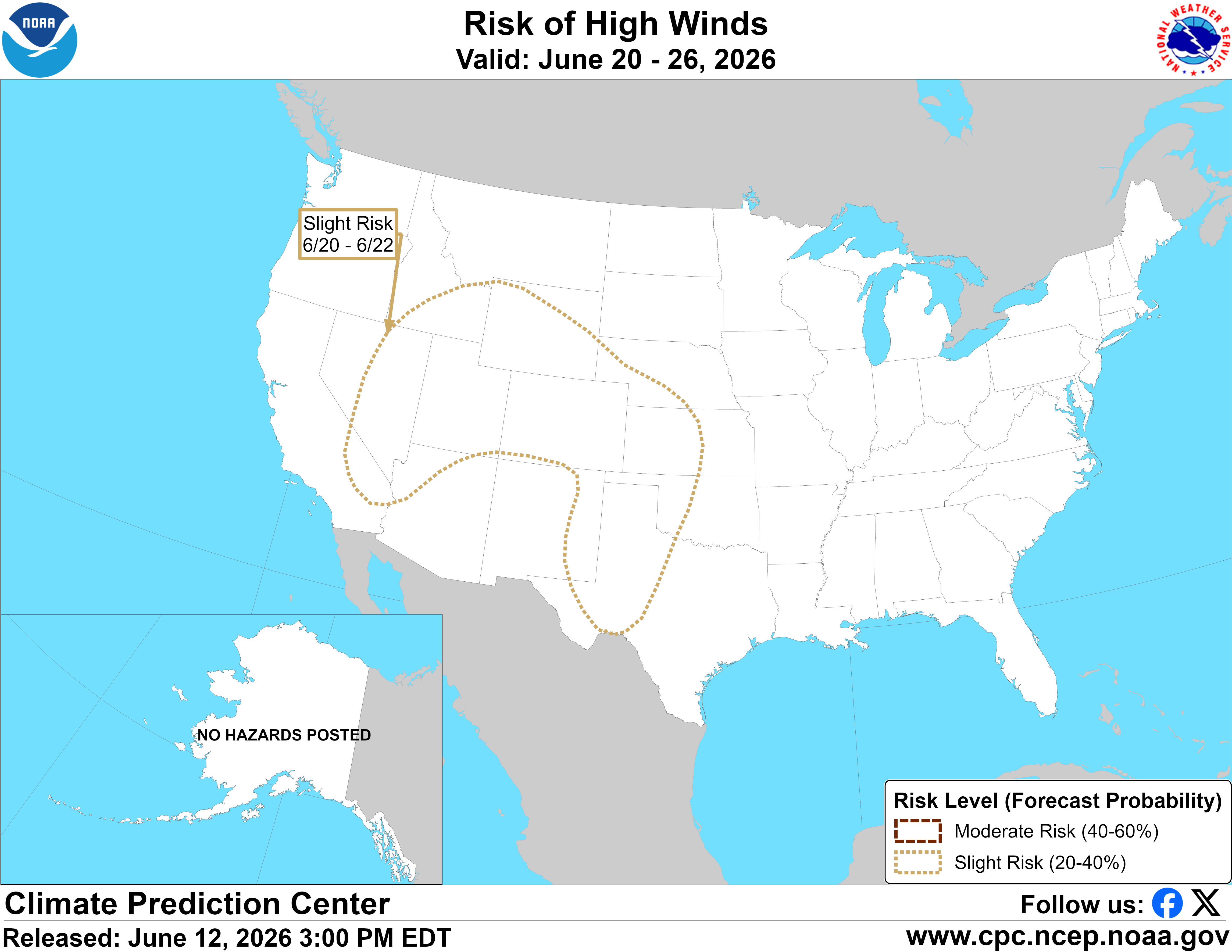Composite Outlook Rules

- All hazards are combined on the composite map which allows users to see the potential for overlapping hazards and multiple time windows within the 8-14 day period.

NOTE: Only moderate and high risk are included on the static composite map while all risk categories are displayed on the interactive map.

- The composite map will also highlight areas of possible flooding and additional frozen precipitation that does NOT fall into the heavy snow category (i.e. freezing rain).

Hazardous Temperature Outlook Rules

- Highlights areas with a slight (20%)moderate (40%) and high (60%) risk of temperatures that are deemed hazardous.

- Much Below Normal = Daily minimum temperatures less than the 15th percentile and near or less than 32°F.

- Much Above Normal = Daily maximum temperatures >85th percentile and 90°F or greater, or minimum temperatures greater than 80°F.

- During the wind chill season (October - March) areas at risk of low wind chills may be outlined.

- During the excessive heat season (April - September) areas at risk of high heat indices may be outlined.

- All percentiles are in comparison to the 1991-2020 climate normal.

Heavy Precipitation Outlook Rules

- Highlights areas with a slight (20%)moderate (40%) and high (60%) risk of heavy precipitation in the 8-14 day time window.

- Heavy precipitation is defined as a 3-day total precipitation amount exceeding the 85th percentile and greater than 1 inch for the location and time of year.

- All percentiles are in comparison to the 1991-2020 climate normal.

Heavy Snow Outlook Rules

- Highlights areas with a slight (20%)moderate (40%) and high (60%) risk of heavy snowfall in the 8-14 day time window.

- Heavy snow is defined as a 3-day total liquid equivalent precipitation amount exceeding the 85th percentile and greater than 0.5 inches for the location and time of year with temperatures that are conducive to snow.

- All percentiles are in comparison to the 1991-2020 climate normal.

High Wind Outlook Rules

- Highlights areas with a slight (20%)moderate (40%) risk of sustained wind speeds exceeding the 85th percentile and at least 25-50 mph at any time over a 3-day period

NOTE: There is no high risk category for high winds.

- All percentiles are in comparison to the 1991-2020 climate normal.

Rapid Onset Drought Methodology ***Experimental***

- Rapid Onset Drought Products are experimental at this time and can be found in the 8-14 Day Composite Maps and 8-14 Day Interactive map

- This new product uses initial conditions such as antecedent dryness, and temperature and precipitation outlooks during the next 2 weeks to communicate the risk of rapidly developing drought during the next 2-3 weeks.

- The primary tools used to define areas of rapidly developing drought are: 1) Abnormal Dryness (D0 on the current U.S. Drought Monitor), 2) Soil moisture below the 30th percentile (very dry soil), 3) 7-day positive temperature anomalies (warmer than normal temperatures) from the National Digital Forecast Database, 4) No precipitation forecast or forecast negative precipitation anomalies (no precipitation or below normal precipitation) from the Weather Prediction Center (WPC) and the CPC's 8-14 day outlooks, 5) Warm and/or dry conditions favored in the Week-2 outlook.

6-10 Day Heat Index Outlook

6-10 Day Wind Chill Outlook

8-14 Day Heat Index Outlook

8-14 Day Wind Chill Outlook

Heat Index Outlook Rules

Wind Chill Outlook Rules

6-10 Day Heat Index Outlook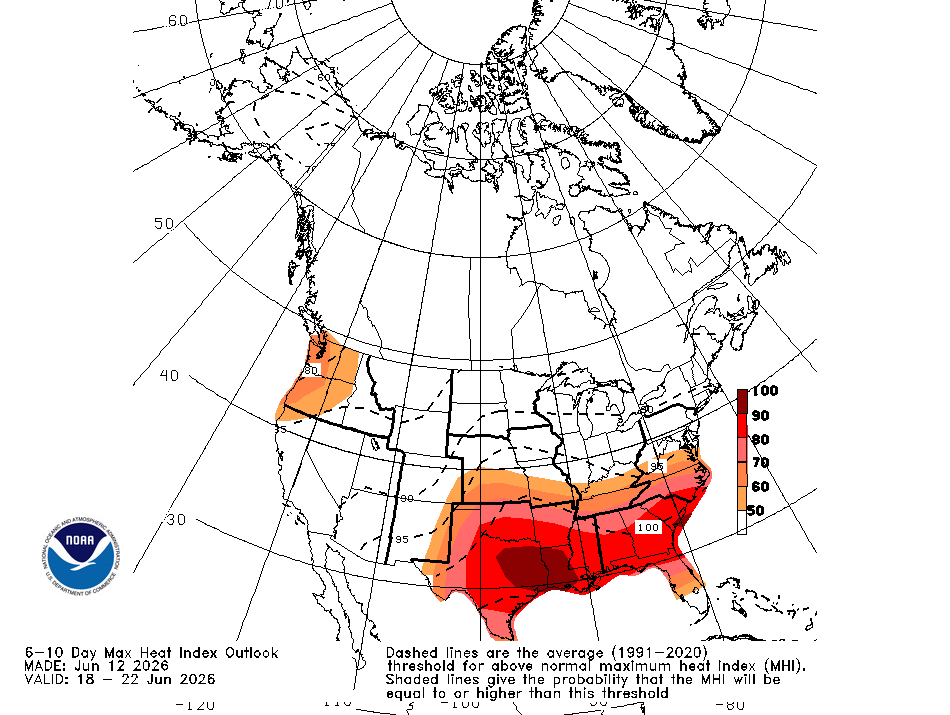6-10 Day Wind Chill Outlook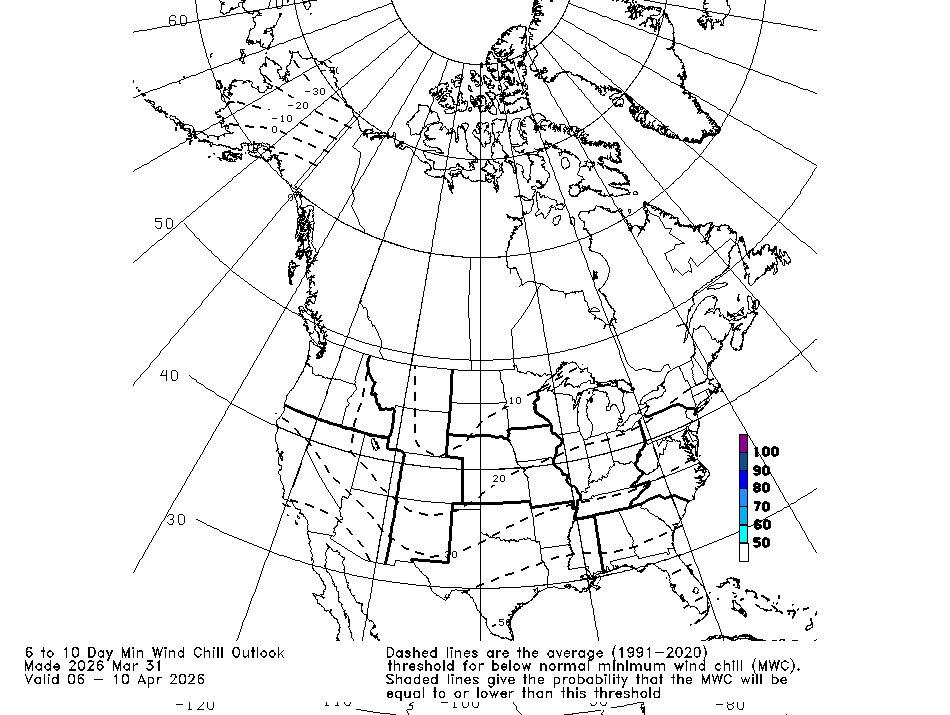8-14 Day Heat Index Outlook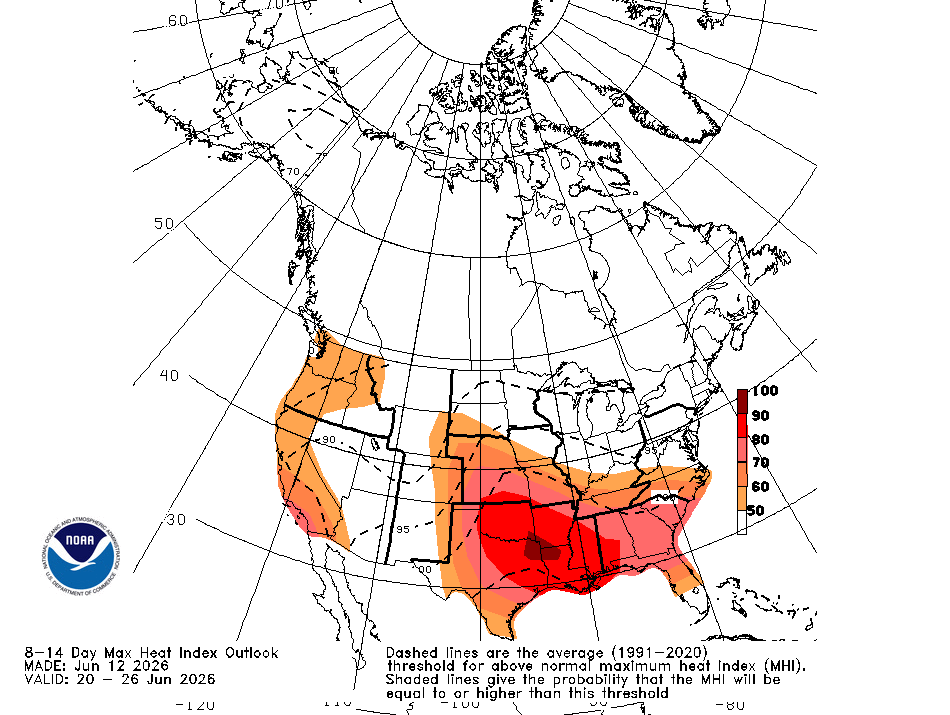8-14 Day Wind Chill Outlook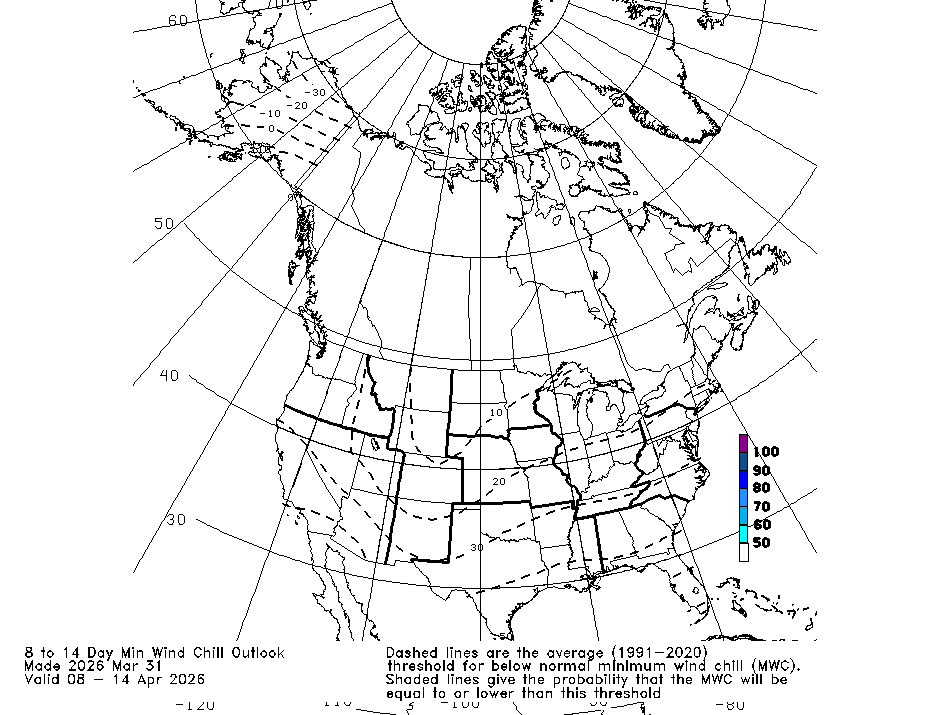Heat Index Outlook Rules

Issued from April 1st through September 30th

- Provides users with the probability that the heat index will be above the 1991-2020 average threshold for above-normal maximum heat index in the time range for a given location.

- Dashed lines show the threshold for above-normal maximum heat index.

- The shaded areas depict the probability of heat index higher than the threshold for above-normal maximum heat index during the forecast period.

Wind Chill Outlook Rules

Issued from October 1st through March 31st.

- Provides users with the probability that the wind chill will be below the 1991-2020 average threshold for below-normal minimum wind chill in the time range for a given location.

- Dashed lines show the threshold for below-normal minimum wind chill.

- The shaded areas depict the probability of wind chill lower than the threshold for below-normal minimum wind chill during the forecast period.

Drought Monitor

Monthly Drought Outlook

Seasonal Drought Outlook

Drought Monitor Intensity Designations

Drought Trends

Drought MonitorDrought Monitor Intensity Designations

D0 (Abnormally Dry)

- Going into drought: short-term dryness slowing planting, growth of crops or pastures.

- Coming out of drought: Some lingering water deficits; pastures or crops not fully recovered.

D1 (Moderate Drought)

- Some damage to crops, pastures; streams, reservoirs or wells low; some water shortage developing or imminent voluntary water use restrictions requested.

D2 (Severe Drought)

- Crop or pasture losses likely; water shortages common; water restrictions imposed.

D3 (Extreme Drought)

- Major crop/pasture losses; widespread water shortages or restrictions.

D4 (Exceptional Drought)

- Exceptional and widespread crop/pasture losses/fire risk; shortage of water in reservoirs, streams and wells creating water emergencies.

Monthly Drought Outlook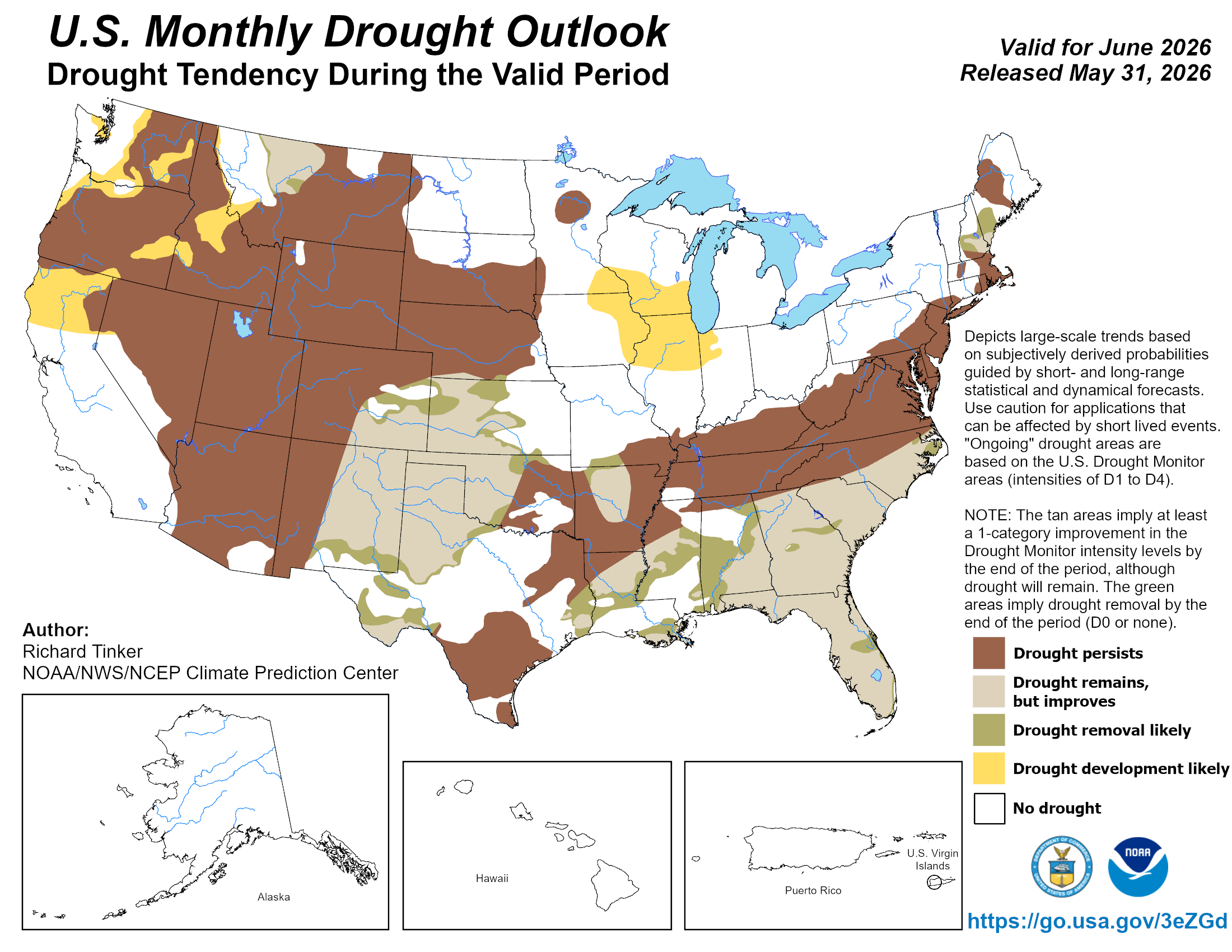Seasonal Drought Outlook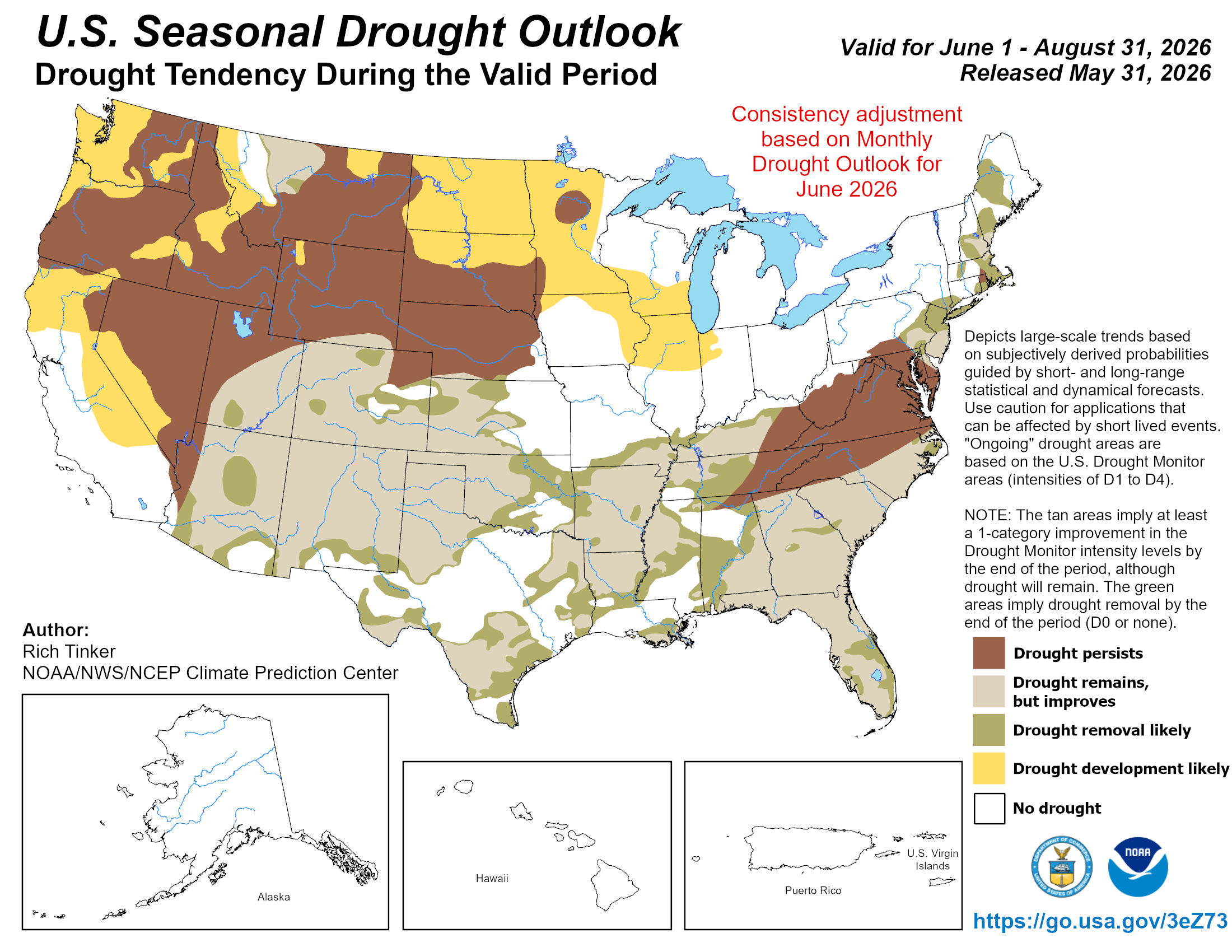The maps above show forecast drought conditions that are broken down into four categories:

1) Drought Persists (brown)

2) Drought remains but improves (i.e. going from D2 to D1) (peach)

3) Drought removal is likely (green)

4) Drought Development is likely (yellow)

Tropical Hazards and Benefits Outlook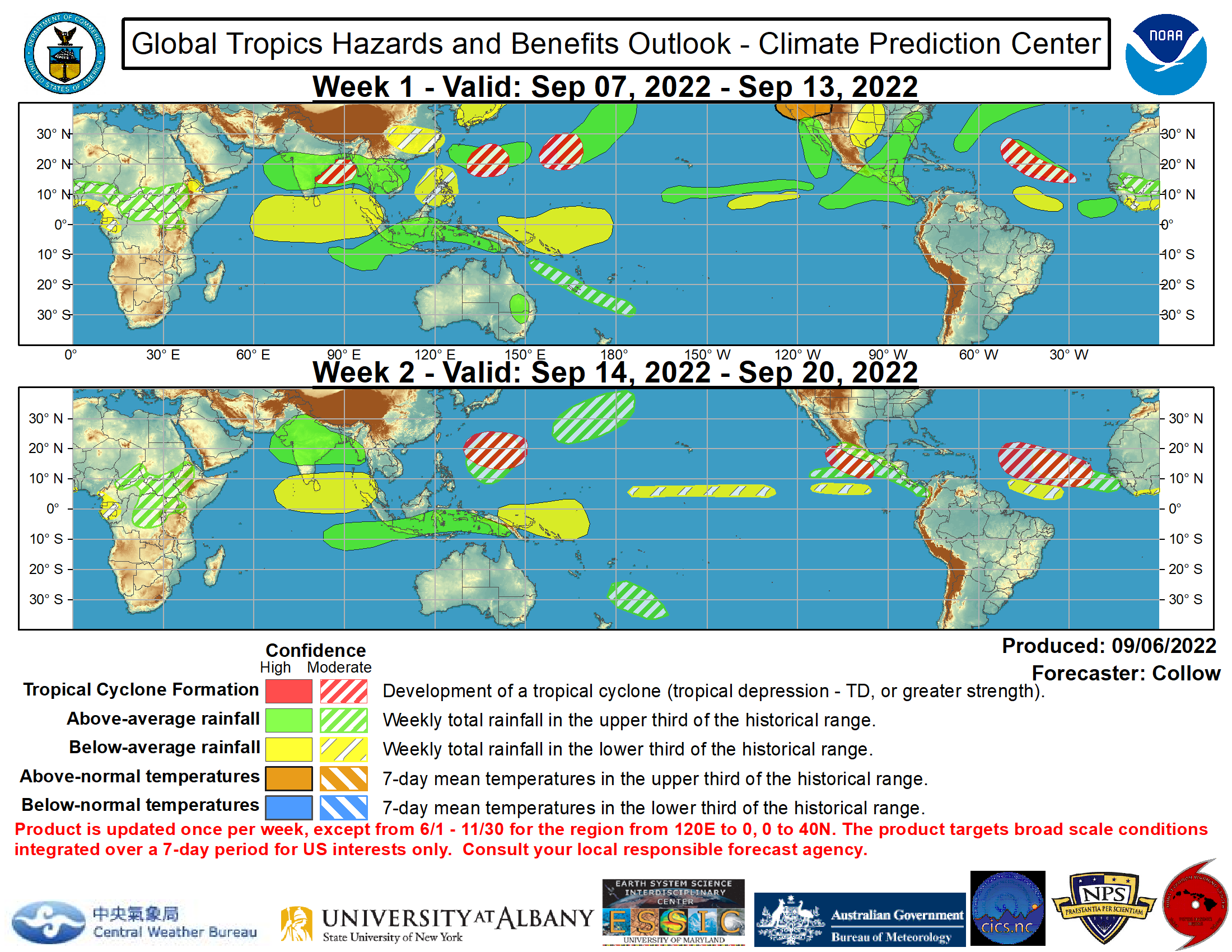Logistics

- Outlook Period: Week 1 and Week 2.